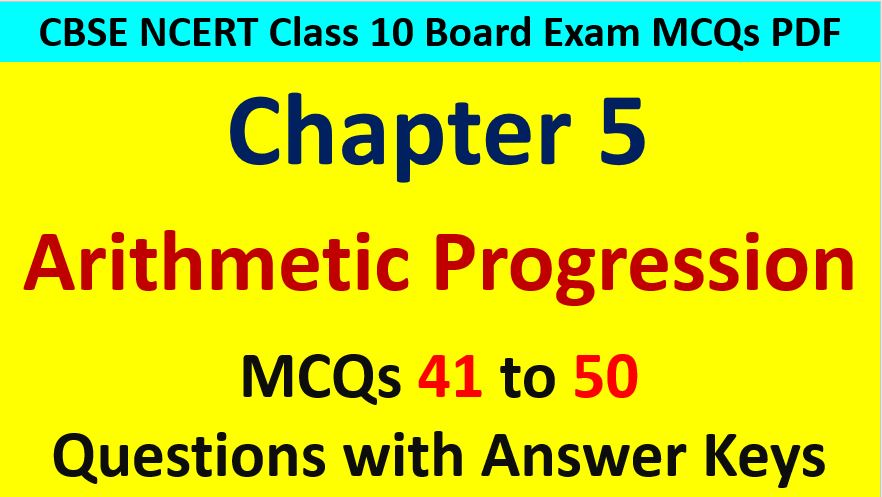Friday, October 22, 2021
Home > CBSE Class 10 > Arithmetic Progression CBSE Class 10 MCQ Questions with Answers Keys

# Arithmetic Progression CBSE Class 10 MCQ Questions with Answers KeysHi students, Welcome to AMBiPi (Amans Maths Blogs). In this article, you will get Arithmetic Progression CBSE Class 10 MCQ Questions with Answer Keys. You can download this PDF and save it in your mobile device or laptop etc.

Arithmetic Progression CBSE Class 10 MCQ Question No 41:

If p, q, r are in AP, then p3 + r3 – 8q3 is

Option A : 4pqr

Option B : -6pqr

Option C : 2pqr

Option D : 8pqr

Option B : -6pqr

Arithmetic Progression CBSE Class 10 MCQ Question No 42:

Two APs have same common difference. The first term of one of these is -1 and that of the other is -8. Then, the difference between their 4th term is

Option A : -1

Option B : -8

Option C : 7

Option D : -9

Option C : 7

Arithmetic Progression CBSE Class 10 MCQ Question No 43:

If (p – 1), (p + 3) and (3p – 1) are in AP, then p is equal to

Option A : 0

Option B : 2

Option C : 4

Option D : 6

Option C : 4

Arithmetic Progression CBSE Class 10 MCQ Question No 44:

If the common difference of an AP is 3, then find the value of a20 – a15.

Option A : 5

Option B : 3

Option C : 15

Option D : 20

Option C : 15

Arithmetic Progression CBSE Class 10 MCQ Question No 45:

In an AP, if d = -2, n = 5 and an = 0, then find the value of a is

Option A : 10

Option B : 5

Option C : -8

Option D : 8

Option D : 8

Arithmetic Progression CBSE Class 10 MCQ Question No 46:

The common difference of the AP 1/p, (1 – p)/p, (1 – 2p)/p, … is

Option A : p

Option B : -p

Option C : -1

Option D : 1

Option C : -1

Arithmetic Progression CBSE Class 10 MCQ Question No 47:

If the nth term of an AP is (2n + 1), then the sum of first three terms is

Option A : 6n + 3

Option B : 15

Option C : 12

Option D : 21

Option B : 15

Arithmetic Progression CBSE Class 10 MCQ Question No 48:

If the sum of the first n terms of an AP is An + Bn2, where A and B are constant, the common difference of the AP will be

Option A : A + B

Option B : A – B

Option C : 2A

Option D : 2B

Option D : 2B

Arithmetic Progression CBSE Class 10 MCQ Question No 49:

An AP consists of 31 terms. If its 16th term is m, the sum of all the terms of this AP is

Option A : 16m

Option B : 47m

Option C : 31m

Option D : 52m

Option C : 31m

Arithmetic Progression CBSE Class 10 MCQ Question No 50:

If Sn denote the sum of first n terms of an AP and if S2n = 3Sn, then find the ratio of S3n : Sn.

Option A : 4

Option B : 6

Option C : 8

Option D : 10